GATE  >  Test: Solid State Physics - 1

# Test: Solid State Physics - 1

Test Description

## 20 Questions MCQ Test GATE Physics Mock Test Series | Test: Solid State Physics - 1

Test: Solid State Physics - 1 for GATE 2023 is part of GATE Physics Mock Test Series preparation. The Test: Solid State Physics - 1 questions and answers have been prepared according to the GATE exam syllabus.The Test: Solid State Physics - 1 MCQs are made for GATE 2023 Exam. Find important definitions, questions, notes, meanings, examples, exercises, MCQs and online tests for Test: Solid State Physics - 1 below.
Solutions of Test: Solid State Physics - 1 questions in English are available as part of our GATE Physics Mock Test Series for GATE & Test: Solid State Physics - 1 solutions in Hindi for GATE Physics Mock Test Series course. Download more important topics, notes, lectures and mock test series for GATE Exam by signing up for free. Attempt Test: Solid State Physics - 1 | 20 questions in 40 minutes | Mock test for GATE preparation | Free important questions MCQ to study GATE Physics Mock Test Series for GATE Exam | Download free PDF with solutions
 1 Crore+ students have signed up on EduRev. Have you?
*Answer can only contain numeric values
Test: Solid State Physics - 1 - Question 1

### The density of copper (Cu) is 8.90 g/m3 with contribution of 1 electron/atom. The plasma frequency of copper is_______x 1016 /s. (Given atomic weight = 63.5)(Round off to one decimal place)

Detailed Solution for Test: Solid State Physics - 1 - Question 1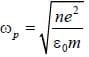n = concentration of free electron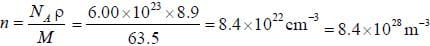and for electron,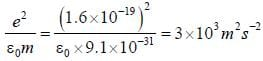⇒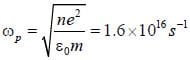*Answer can only contain numeric values
Test: Solid State Physics - 1 - Question 2

### Assume that E vs k relationship of e- in conduction band ofliypothetical tetravalent n-type semiconductor can be expressed by E = ak2 + constant The cyclotron resonance for e- in a field B = 0.1 wb/m2 occurs at ωe = 1.8 x  1011 rad/s The value of a =________x 10-38 J. m2 (Round off to one decimal place)

Detailed Solution for Test: Solid State Physics - 1 - Question 2

⇒ An e- in conduction band behave as if it had an effective mass m* given by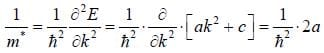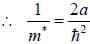and cyclotron resonance frequency for eis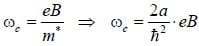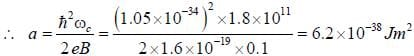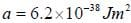*Answer can only contain numeric values
Test: Solid State Physics - 1 - Question 3

### The maximum wavelength of photon to break a cooper pair in Lead which has energy gap of 2.73 meV is_____________x 105 nm. (Round off to three decimal places)

Detailed Solution for Test: Solid State Physics - 1 - Question 3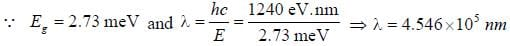*Answer can only contain numeric values
Test: Solid State Physics - 1 - Question 4

The lattice constant of unit cell of BCC structure α - Iron is 0.288 nm. The number of atoms/mm2 in the plane of (1 0 0) is_____x 1012 atom/mm2. (Answer should be an integer)

Detailed Solution for Test: Solid State Physics - 1 - Question 4

The number of atoms in unit cell = 2 Therefore, the atomic density in (100) plane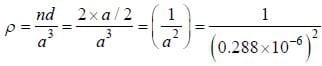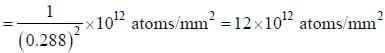*Answer can only contain numeric values
Test: Solid State Physics - 1 - Question 5

For a sphere of radius x, polarised along the radius vector such that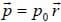. The total charge is     (Answer should be integer).

Detailed Solution for Test: Solid State Physics - 1 - Question 5

Total charge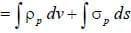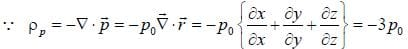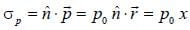Thus, equation (i) implies
Total charge = -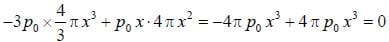Test: Solid State Physics - 1 - Question 6

The metals an oppaque to light for which

Detailed Solution for Test: Solid State Physics - 1 - Question 6

The defractive index η =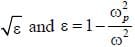If light of frequency ω < ωp  incident on a metal of plasma frequency ωp. than η is imaginary. This mean that such light cannot transmitted through the metal but is totally reflected from its surface.
∵ ωp > ω ⇒ ε → -ve ⇒ η → imaginary
∴Thus metal is opaque to light for which ω < ωp

Test: Solid State Physics - 1 - Question 7

Optical excitation of intrinsic Germanium creates an average density of 1018 conduction electrons per m3 in the material at liquid nitrogen temperature. At this temperature electrons and holes mobilities are equal.
μ = 0.5 x 104cm2 /volts sec and Germanium dielectric constant is 20. The diffusion coefficient for electron is

Detailed Solution for Test: Solid State Physics - 1 - Question 7

The diffusion coefficient D is given by Einstein relation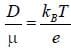∵ liquid nitrogen temperature TD = 77K . μ = 0.5 x 10-4 x 104 m2 /V.s = 0.5 m2/V.s
e = 1.6 x 10-19
kB =1.38 x 10-23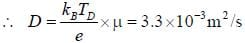Test: Solid State Physics - 1 - Question 8

In Raman effect

Test: Solid State Physics - 1 - Question 9

An ac current of frequency 1 GHz is observed through Josephson junction, the applied dc voltage is

Detailed Solution for Test: Solid State Physics - 1 - Question 9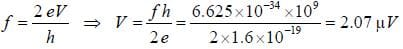Test: Solid State Physics - 1 - Question 10

The polarizability 'α' of an e- moving in a circular orbit of radius ‘r’ about charge +e in a field directed at light angle to plane of orbit is

Detailed Solution for Test: Solid State Physics - 1 - Question 10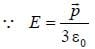.....(i)
Dipole moment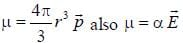∴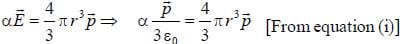∴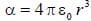*Answer can only contain numeric values
Test: Solid State Physics - 1 - Question 11

The density of states for electron hi a metal is given by D  (E)dE = 13.6x 1027 E1/2 dE and ρ = 8.5 x 1028 /m3. The energy of Fermi level is___________eV. (Round off to three decimal places)

Detailed Solution for Test: Solid State Physics - 1 - Question 11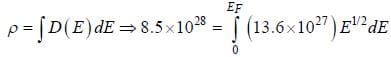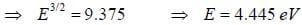*Answer can only contain numeric values
Test: Solid State Physics - 1 - Question 12

For lead the superconductivity occurs at 7.19 k when there is zero applied magnetic field. When the magnetic field 0.074 T is applied at temperature 2.0 k superconductivity will stop. The magnetic field that should be
applied so that superconductivity will not occur at any temperature is___________x 10-3 T. (Answer should
be an integer).

Detailed Solution for Test: Solid State Physics - 1 - Question 12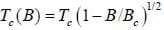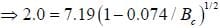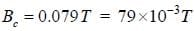*Answer can only contain numeric values
Test: Solid State Physics - 1 - Question 13

The energy of the conduction band at which the probability of the conduction electrons state will occupied by
election is 90% at T = 800K. is__________(eV). [Given: EF =5.52eV]
(Round off to two decimal places)

Detailed Solution for Test: Solid State Physics - 1 - Question 13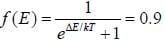.......(i)
Now T = 800 K, now solving equation (i) for ΔE, we get ΔE = 5.42 eV
We get, ΔE = 5.42eV

Test: Solid State Physics - 1 - Question 14

Calculate the relative permeability of material having magnetization of 3400 ampere/meter and flux density of 0.0045 wb/m2.

Detailed Solution for Test: Solid State Physics - 1 - Question 14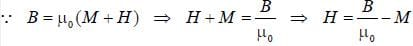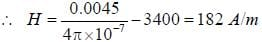also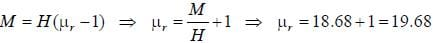Test: Solid State Physics - 1 - Question 15

A long thin uniformly magnetized needle of hard steel length 12 cm is suspended by a torsionless suspension and makes free, small angle oscillations about an axis through the center in a honzontal plane in the earth's magnetic field. The period of oscillation is four seconds. The density of magnetization of steel is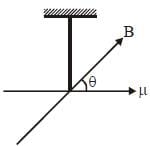Detailed Solution for Test: Solid State Physics - 1 - Question 15

Let μ be the magnetic moment of the steel needle. The potential energy in the earth’s magnetic field.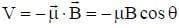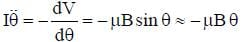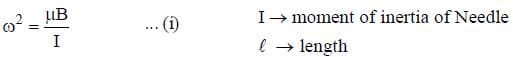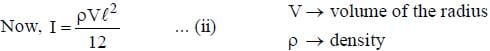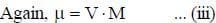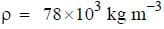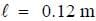T = 4s
B = 5 x 10 -5 wm-2
M → Magnetization
Using (i), (ii) and (iii)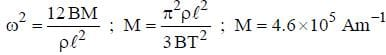Test: Solid State Physics - 1 - Question 16

The energy of interaction of two atoms at a distane 'r' apait can be written as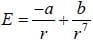where, a, b are constants
If two atoms are pulled apart show that they will separete most easily when

Detailed Solution for Test: Solid State Physics - 1 - Question 16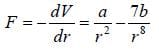The particles will separate most easily when force F is minimum. Now, F to be minimum,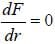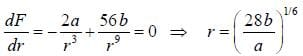Test: Solid State Physics - 1 - Question 17

Consider a crystal containing a paramagnetic impurity whose energy level are in a given magnetic field. The specific heat of the crystal at high temperature will vary with

Detailed Solution for Test: Solid State Physics - 1 - Question 17

The contribution of impurity to the partition function of the crystal is z =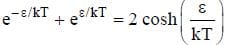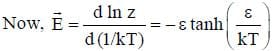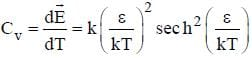At high temperature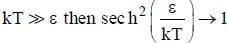Thus,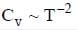Test: Solid State Physics - 1 - Question 18

A rough sketch of the absorbtion co-efficient for the EM radiation for a non magnetic pure solid substance. The solid absorb light in infrared (0.15 eV) and then does not absorb until the photon energy is 2.3 eV. The minimum number of atoms per unit cell in the crystal should be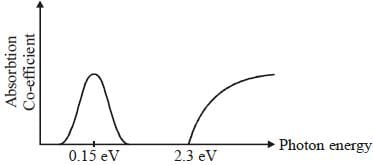Detailed Solution for Test: Solid State Physics - 1 - Question 18

There are minimum more than 2 atoms per unit cell otherwise the optical branch would have been absent and there would be no absorbtion peak at 0.15 eV.

Test: Solid State Physics - 1 - Question 19

X-rays are reflected from a simple cubic crystal by Bragg reflection. If the density of the crystal is measured with r.m.s. error of 3 parts in 104 and if the angle made by the incident and reflected ray make with the crystal plane is 6° and is measured with an r.m.s. error 3.4 minutes of arc then what is the error in the determination of wavelength.

Detailed Solution for Test: Solid State Physics - 1 - Question 19

For simple cubic crystal n = 1.
For first order reflection 2d sin θ = λ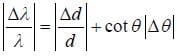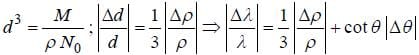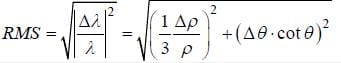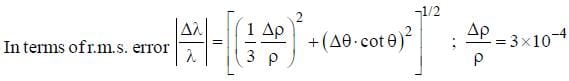∵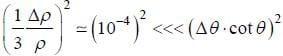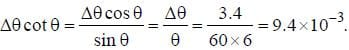Test: Solid State Physics - 1 - Question 20

The basis vectors of a lattice is given by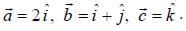The volume of the reciprocal lattice are given by

Detailed Solution for Test: Solid State Physics - 1 - Question 20

Volume ot unit cell in direct lattice.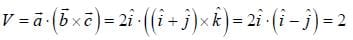Therefore, basic vectors of reciprocal lattice are given by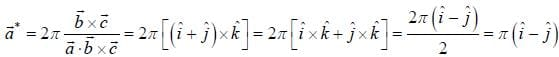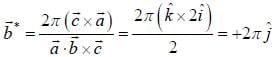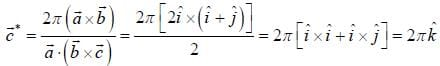Therefore, volume of unit cell in reciprocal Lattice.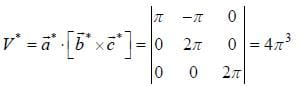## GATE Physics Mock Test Series

1 docs|34 tests
 Use Code STAYHOME200 and get INR 200 additional OFF Use Coupon Code
Information about Test: Solid State Physics - 1 Page
In this test you can find the Exam questions for Test: Solid State Physics - 1 solved & explained in the simplest way possible. Besides giving Questions and answers for Test: Solid State Physics - 1, EduRev gives you an ample number of Online tests for practice

1 docs|34 tests